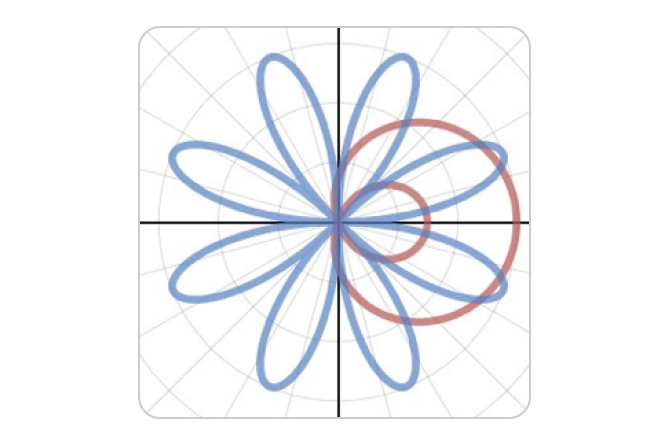# Polar Graphing

Convert the coordinate plane to a polar grid with just a pair of clicks, then you’re free to explore the beauty of circles, spirals, roses, limacons and more in this polar graphing playground. Get started with the video on the right, then dive deeper with the resources and challenges below.

## Getting Started

The Desmos Graphing Calculator considers any equation or inequality written in terms of $$r$$ and $$𝜃$$ to be in polar form and will plot it as a polar curve or region.

By default, polar curves are plotted for values of $$𝜃$$ in the interval $$[0, 12π].$$ If the calculator is able to detect that a curve is periodic, its default domain may be adjusted so that one full period is plotted. In any case, it is possible to set the plot’s domain manually using the inputs beneath the expression.

Polar inequalities will only display regions where the radius is positive (to avoid many common cases, like $$r\lt 1$$, where including regions of negative radius would confusingly shade the whole plane). If you want your inequality to include regions with negative radius, simply use its absolute value.If you would like to display a polar grid, rather than the default Cartesian grid, that option is available in the Graph Settings menu, accessible by clicking the wrench icon in the upper right-hand corner of the graph paper.

Note that polar equations must be linear in $$r$$, and it is currently not possible to plot polar equations of the form $$𝜃 = f(r).$$## Polar Graphing in Action

### "The best way to learn is to do." – Paul Halmos### Graphing Challenges

Stretch your polar skills with graphing challenges.